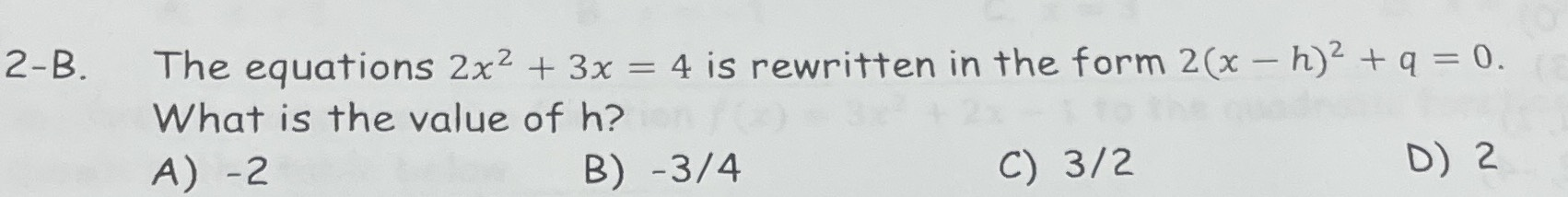### ¿Todavía tienes preguntas de matemáticas?

Pregunte a nuestros tutores expertos
Algebra
Pregunta2-B. The equations $$2 x ^ { 2 } + 3 x = 4$$ is rewritten in the form $$2 ( x - h ) ^ { 2 } + q = 0$$ . What is the value of $$h$$ ?

A) $$- 2$$ B) $$- 3 / 4$$ C) $$3 / 2$$ D) $$2$$